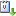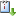Usted está aquí: "The Brezis-Nirenberg problem revisited"

# "The Brezis-Nirenberg problem revisited"

Andrzej Szulkin (Universidad de Estocolmo) - jueves, 27 de octubre del 2011, 11 hrs.
Ponente:
Cuándo 27/10/2011 de 11:00 a 12:00 Sala de seminarios Graciela SalicrupvCaliCal

We consider the celebrated problem of Brezis and Nirenberg

$-\Delta u-\lambda u = |u|^{2^*-2}u \text{ in } \Omega, \quad u=0 \text{ on } \partial\Omega,$

where $\Omega\subset\mathbb{R}^N$ ($N\ge 4$) is a bounded domain and $2^*:=2N/(N-2)$ is the critical Sobolev exponent. Denote the $m$-th eigenvalue of $-\Delta$ with zero Dirichlet boundary data by $\lambda_m$. After reviewing some known results we show that this problem has a ground state solution $u$ for all $\lambda\in\mathbb{R}$ ($\lambda\ne \lambda_m$ if $N=4$). We also show that the Morse index of $u$ is $m+1$ if $\lambda\in [\lambda_m,\lambda_{m+1})$ and use this fact to study the nodal properties and symmetry of ground states. This is joint work with Tobias Weth and Michel Willem.